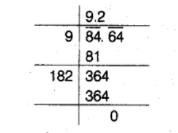# Find the decimal fraction,

Question:

Find the decimal fraction, which when multiplied by itself, gives 84.64.

Solution:

Let the decimal fraction be $\boldsymbol{x}$.

When a decimal fraction is multiplied by itself, then product $=x \times x=x^{2}$

But product $=84.64$ [given]

$\therefore \quad x^{2}=84.64$

$\Rightarrow \quad x=\sqrt{84.64}$

Now, piace the bar over the numbers, then square root is given below.$\therefore \quad x=\sqrt{84.64}=9.2 .$

Hence, the required decimal is $9.2$.